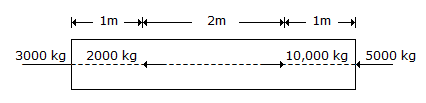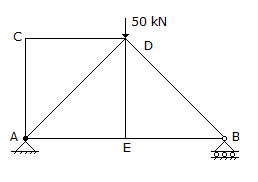# Civil Engineering - UPSC Civil Service Exam Questions

21.

The role of superplasticizer in a cement paste is to

 A. disperse the particles B. disperse the particles and to remove air bubbles C. disperse the particles, remove air bubbles and to retard setting D. retard setting

Explanation:

No answer description available for this question. Let us discuss.

22.

For stability analysis of slopes of purely cohesive soils, the critical centre is taken to lie at the intersection of

 A. the perpendicular bisector of the slope and the locus of the centre B. the perpendicular drawn at one-third slope from the toe and the locus of the centre C. the perpendicular drawn at two-third slope from the toe and the locus of the centre D. directional angles

Explanation:

No answer description available for this question. Let us discuss.

23.

A prismatic bar of uniform cross-sectional area of 5 cm2 is subjected to axial loads as shown in the given figure. Portion BC is subjected to an axial stress ofA. 400 kg/cm2 tansion, B. 2000 kg/m2 compression C. 1000 kg/cm2 tension D. 600 kg/cm2 tension

Explanation:

No answer description available for this question. Let us discuss.

24.

Which one of the following methods would give accurate results in determining the direction of the observer's meridian ?

 A. Observation of circumpolar stars on the same vertical B. Observation of circumpolar stars at culmination C. Extra-meridian observation of a circumpolar star D. Observation of the sun at equal altitudes

Explanation:

No answer description available for this question. Let us discuss.

25.

In a truss work as shown in the figure given above, what is the force induced in the member DE ?A. 50 kN (tensile) B. zero C. 50 kN (compressive) D. 25 kN (compressive)